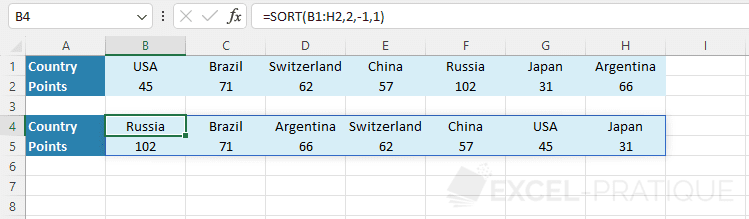# Excel Function: SORT

The Excel function SORT sorts the contents of a range of cells or a table and returns a sorted table based on the defined parameters (or by default).

Usage:

`=SORT(array)`

or

`=SORT(array, sort_index, sort_order, by_column)`

## Default Sorting

In this example, the goal is to sort the table based on the country name: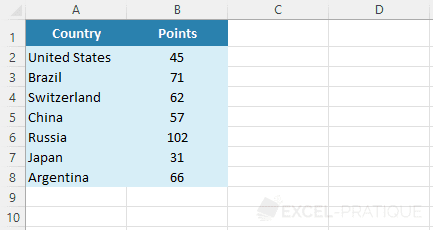Select the SORT function and simply enter the cell range of the table to perform the default sort (ascending sort based on the first column of the table):

``=SORT(A2:B8)``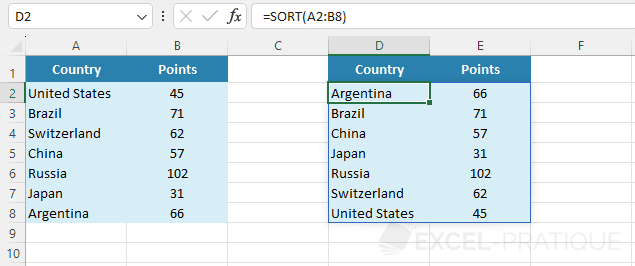## Sorting Based on Another Column

To sort the table based on the points (thus based on the second column), enter 2 as the second argument:

``=SORT(A2:B8,2)``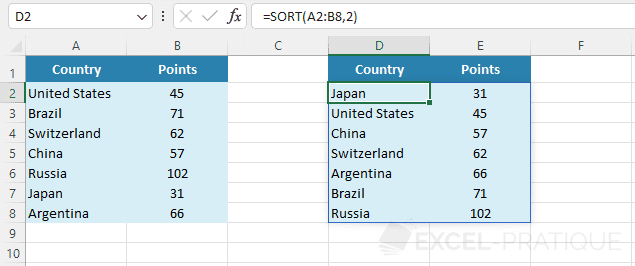## Sorting in Descending Order

To achieve a descending sort this time, enter -1 as the third argument:

``=SORT(A2:B8,2,-1)``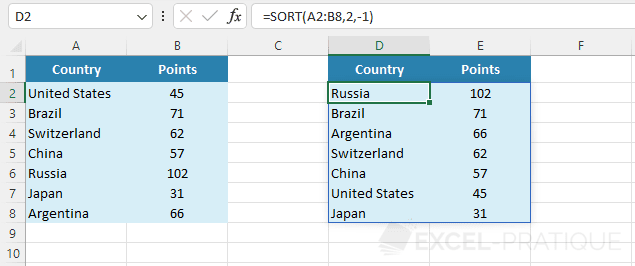## Sorting by Column

The last optional argument allows for sorting by column (instead of sorting by row) by entering the value 1 (or TRUE):

``=SORT(B1:H2,2,-1,1)``